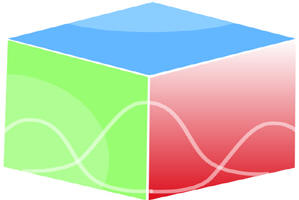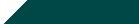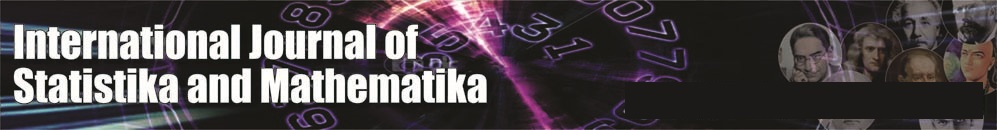``Home| Journals | Statistics Online Expert | About Us | Contact UsUntitled Document

[

International Journal of Statistika and Mathematika, ISSN: 2277- 2790 E-ISSN: 2249-8605

Volume 9, Issue 2, March 2014 pp 56-60

Research Article

Transformed Variable ARMA Model

P. Ramakrishna Reddy, B. Sarojamma

Department of Statistics, S.V. University, Tirupati – 517502, Andhra Pradesh, INDIA.

Academic Editor: Dr. Dase R. K.

Abstract

There are many forecasting models like moving average  MA (1), MA (2), weighted moving average, multiple moving average, simple exponential smoothing, double exponential smoothing, triple exponential smoothing, adaptive smoothing, auto regression AR (1), AR (2), ARMA, ARIMA, ARCH, GARCH, etc. In this paper we discussed about transformed variable ARMA models. ARMA model is a combination of auto regression with moving averages. Generally auto regression and moving averages are calculated with time series values. By taking log values and geometric mean ARMA model values smoothing the data. Arithmetic mean effects the extreme values where as geometric mean cannot effects extreme values as much as A.M.   By taking logarithm and geometric mean transformations to the time series observations to perform ARMA models, we get logarithm ARMA model and geometric mean ARMA model. Logarithm ARMA model and geometric mean ARMA model are tested for goodness of fit by using Kolomogrov-Smirnov test. Mean Square Error (MSE) criterion is used for choosing best model among ARMA (1, 1), Logarithmic ARMA (1, 1) and geometric mean ARMA (1, 1) models.